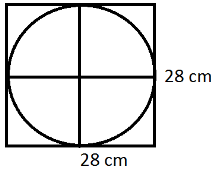QuestionAnswers

# Find the area of a circle inscribed in a square of side 28 cm as shown in figure.Hint: When a circle is inscribed in a square the diameter of the circle is equal to the side length of the square.
You can find the perimeter and area of the square, when at least one measure of the circle or the square is given.
For a square with side length S, the following formulas are used
Perimeter $= 4S$
Area $= {S^2}$
Diagonal $= S\sqrt 2$
For a circle write the radius r, the following formulas are used circumference $= 2\pi r$
Area $= \pi {r^2}$
Therefore,

As we know before the diameter of the circle is equal to the side length of the square.
The side length of the square $= 28\,cm$
Area of a circle $= \pi {r^2}$
To find the area first of all we find the radius of the circle.
$d = 28\,cm\, =$side length of the square
Where ‘d’ is diameter of the circle
$r = \dfrac{d}{2} = \dfrac{{28}}{2} = 14\,cm$
As we know that radius is half of the diameter
Now we have the value of ‘r’ that is 14 cm
Putting the value of r in the formula
Area of circle
$= \pi {r^2}$
$= \pi \times {(14)^{2\,}}\,c{m^2}$
$= 196\,\pi \,c{m^2}$
Hence the area of the circle is $196\,\pi \,c{m^2}$

Note: If they ask to find the area of the square, we used the formula
Area of the square $= {S^2}$
$S = 28\,cm$
$= 28 \times 28\,c{m^2}$
$= 784\,c{m^2}$
View Notes
Area of a Sector of a Circle FormulaHow to Find Square Root of a NumberArea of a Rhombus FormulaArea of Square FormulaSurface Area of a Prism FormulaTo Find the Weight of a Given Body Using Parallelogram Law of VectorsThe Making of a ScientistChanging the Period of a PendulumArea of TrapeziumArea of Hollow CylinderImportant Questions for CBSE Class 6 English A Pact with The Sun Chapter 1 - A Tale of Two BirdsImportant Questions for CBSE Class 7 English An Alien Hand Chapter 9 - A Tiger In The HouseImportant Questions for CBSE Class 6 English A Pact with The Sun Chapter 8 - A Pact with the SunImportant Questions for CBSE Class 7 English Honeycomb Chapter 9 - A Bicycle In Good RepairImportant Questions for CBSE Class 6 English A Pact with The Sun Chapter 9 - What Happened to The ReptilesImportant Questions for CBSE Class 6 English Honeysuckle Chapter 8 - A Game of ChanceImportant Questions for CBSE Class 11 Business Studies Chapter 7 - Formation of a CompanyImportant Questions for CBSE Class 6 English A Pact with The Sun Chapter 10 - A Strange Wrestling MatchImportant Questions for CBSE Class 7 English Honeycomb Chapter 2 - A Gift Of ChappalsImportant Questions for CBSE Class 6 English Honeysuckle Chapter 5 - A Different Kind of SchoolCBSE Class 10 Hindi A Question Paper 2020Hindi A Class 10 CBSE Question Paper 2009Hindi A Class 10 CBSE Question Paper 2015Hindi A Class 10 CBSE Question Paper 2016Hindi A Class 10 CBSE Question Paper 2012Hindi A Class 10 CBSE Question Paper 2010Hindi A Class 10 CBSE Question Paper 2007Hindi A Class 10 CBSE Question Paper 2013Hindi A Class 10 CBSE Question Paper 2008Hindi A Class 10 CBSE Question Paper 2014RS Aggarwal Class 8 Mathematics Solutions for Chapter-18 Area of a Trapezium and a PolygonRD Sharma Solutions for Class 9 Maths Chapter 21 - Surface Area and Volume of a SphereRD Sharma Solutions for Class 9 Maths Chapter 19 - Surface Area and Volume of A Right Circular CylinderRD Sharma Solutions for Class 9 Maths Chapter 18 - Surface Area and Volume of a Cuboid and CubeRD Sharma Solutions for Class 9 Maths Chapter 20 - Surface Area and Volume of A Right Circular ConeRD Sharma Class 7 Solutions Chapter 20 - Mensuration I (Area of Circle) (Ex 20.2) Exercise 20.2RS Aggarwal Solutions Class 8 Chapter-18 Area of a Trapezium and a Polygon (Ex 18B) Exercise 18.2NCERT Solutions for Class 9 Maths Chapter 9 Area Of Parrallelograms and triangles In HindiRD Sharma Class 7 Solutions Chapter 20 - Mensuration I (Area of Circle) (Ex 20.1) Exercise 20.1RS Aggarwal Solutions Class 8 Chapter-18 Area of a Trapezium and a Polygon (Ex 18A) Exercise 18.1